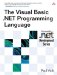# DateInterval Enumeration

 <  Day Day Up  >

 The DateInterval enumeration is used by the DateAdd , DateDiff , and DatePart functions to indicate which part of a date/time value should be used. The DateInterval enumeration values have no constant equivalents, but they do have string equivalents that can be used in place of the enumeration in the overloaded functions. ` Year = 0 ` The year of the date should be used. String equivalent: "yyyy". ` Quarter = 1 ` The quarter of the year (1 through 4) should be used. String equivalent: "q". ` Month = 2 ` The month of the year should be used. String equivalent: "m". ` DayOfYear = 3 ` The day of the year (1 through 366) should be used. String equivalent: "y". ` Day = 4 ` The day of the month should be used. String equivalent: "d". ` WeekOfYear = 5 ` The week of the year (1 through 53) should be used. String equivalent: "ww". ` Weekday = 6 ` The day of the week (1 through 7) should be used. String equivalent: "w". ` Hour = 7 ` The hour of the day should be used. String equivalent: "h". ` Minute = 8 ` The minute of the hour should be used. String equivalent: "n". ` Second = 9 ` The second of the minute should be used. String equivalent: "s".
 <  Day Day Up  >The Visual Basic .NET Programming Language
ISBN: 0321169514
EAN: 2147483647
Year: 2004
Pages: 173
Authors: Paul Vick

Similar book on Amazon